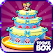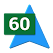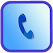## Ed Burg Published Applications :

Strain value Calculator is a good application for calculating strain guage value.
Parallel Resistance Calculation app is simple app that enables computing of parallel resistance very easily.
this is the application for creating sounds like a musical instruments.
This app provides the sounds of catz
Uniform Acceleration value Calculator is a application for calculating Uniform Acceleration value.
Otto Cycle Compression Ratio Calculator is used for finding Otto Cycle Compression Ratio.
This app Consists of Bible Quotes
It is designed to calculate the maximum battery charge time for any given chargable battery, based on the entered information.
this application is used for the calculation of Transferrin serum Iron Saturation
An App to calculate Total Body Water
Beam Deflection Calculator is an simple app for the computation of Beam Deflection.
VCC For Rate of Change is a application for vcc rate calculation
this is the best application for finding Pressure value.This application can produce the currect value.
this is an entertainment application for Money Quote.
this is the application for converting APY value to APR value.
Top ReviewedRecently Reviewed